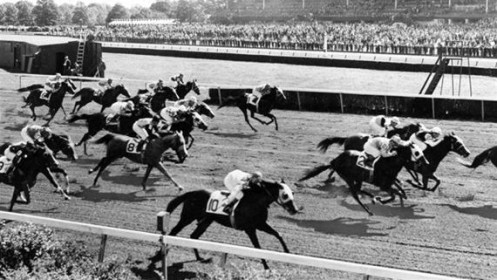In the first week of Jeopardy! tournaments, I keep a running tally of wild card standings. I also provide estimates of each player’s probability of advancing.

How do I do this? With the help of one of my favorite branches of mathematics: combinatorics. Over the next few posts, I’ll introduce you to this useful field.

## What is combinatorics?

According to Wolfram Alpha, combinatorics is “the branch of mathematics studying the enumeration, combination, and permutation of sets of elements and the mathematical relations that characterize their properties.”

In plain English, it helps you figure out how many different ways something can happen.

Let’s say you have to pick 4 people out of a group of 10. How many different ways you can do this?

That all depends on one question: do you care about the order in which they’re selected? Are those four going to be placed in particular spots, or do they just want a ride in your car and don’t care where they sit? That’s the distinction between permutations and combinations. We’ll talk about the first one here.

## Factorial!

Before we get into permutations, however, I need to introduce the concept of factorial.

Let’s start, as usual, with an example completely unrelated to Jeopardy!: horse racing.

Question: Twelve horses start a race. In how many different orders can these horses finish, from first through twelfth?Let’s think about this logically. (For those of you who love technicalities, we’ll assume the horses can’t tie.)

How many horses can finish in first place? Any of them can: 12.

How many horses could then finish in second place? Any of them – except for whatever horse finished in first, because he can’t finish in more than one spot. That means 11 could possibly finish in second given we know the identity of the first-place finisher.

Continuing, 10 can finish in third, 9 in fourth, and so on.

In probability, we multiply the number of individual events to figure out how many total possibilities exist. In this case, there are

 12 × 11 × 10 × 9 × 8 × 7 × 6 × 5 × 4 × 3 × 2 × 1

possible final orders.

Mathematicians have a shortcut for numbers like this: factorial. We call this number 12!, or 12 factorial. (You don’t actually say the number with emphasis or excitement or anything.)

Answer: There are 12! – or 479,001,600 – different possible finishing orders.

A factorial is simply a number multiplied by every single positive integer smaller than it. For example, 3! = 3 × 2 × 1 = 6.

In case you’re more inclined to rules, here’s a formal definition:

 n! = { 1 if n = 0 (n-1)! × n if n ≥ 1

## Permutations: finding the top X finishers

Now, let’s suppose you’ve just read my Fact Primer on parimutuel betting and you’re itching to bet the trifecta: the top three horses, in order. If a trifecta wager costs one dollar, how much would you have to spend to cover every possibility?

Going back to our logical approach, 12 horses could finish first, 11 others could finish second, and 10 others third. That means there are

 12 × 11 × 10

bets, which at one dollar apiece would cost you \$1,320.

When order matters, we refer to such a grouping as a permutation. There are a number of ways to write this, like P(12,3) or 12P3. Aloud, we say “12, choose 3”.

We can use our new friend, factorial, to help us calculate the number of possible permutations. The variable n represents the total number, while k represents the number of spots we’ll choose:

 nPk = n! (n–k)!

In this example we have 12 possible horses (n) from which we want to select the first 3, in order (k). Plugging those into our formula, we get:

 12! = 12! (12-3)! 9!

You can expand that if you like:

 12 × 11 × 10 × 9 × 8 × 7 × 6 × 5 × 4 × 3 × 2 × 1 9 × 8 × 7 × 6 × 5 × 4 × 3 × 2 × 1

With fractions, we can cancel out equal values that appear in both the numerator (above the bar) and the denominator (below the bar):

 12 × 11 × 10 × 9 × 8 × 7 × 6 × 5 × 4 × 3 × 2 × 1 9 × 8 × 7 × 6 × 5 × 4 × 3 × 2 × 1

… which leaves us with 12 × 11 × 10, which matches what we found earlier.

(Note: Using the formal definition of n!, we can also write a shorter definition:

 12 × 11 × 10 × 9! 9!

The 9! in the numerator and the denominator will cancel, leaving 12 × 11 × 10.)

Want to test your skills? Here are a couple of practice problems.

Question 1: In how many different ways can I arrange the six books next to my bed?

Correct response: Show

Question 2: 20 horses start the Kentucky Derby and you want to bet a superfecta (top four in order) on every possible combination. If a superfecta wager costs \$1, how much will you have to spend?

Correct response: Show

In my next post, I’ll discuss combinations: when order does not matter.

1.•